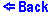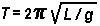The Pendulum
Drag the mass to its new starting position.

This applet shows a pendulum suspended on a 'rigid string'. One can drag the pendulum to it's starting position. Once in motion, the pendulum can be 'caught' by clicking and holding the mass when it has reached it's maximum angle. Thus, the pendulum can be brought to it's new starting position. The experimental period is shown in the panel above the pendulum itself and is obtained by multiplying the time needed to make half an oscillation by two. The theoretical period, on the other hand, is obtained by a formula which is valid only for small angles., i.e,where T is the period of the oscillations, L is the length of the string and g is the acceleration due to gravity.

Thus, as the initial angle is larger one can notice a dramatic difference in the two periods.